# COMP 171 Trees Binary Trees and Binary Search

• Slides: 37COMP 171 Trees, Binary Trees, and Binary Search Trees2 Trees * Linear access time of linked lists is prohibitive n Does there exist any simple data structure for which the running time of most operations (search, insert, delete) is O(log N)? * Trees Basic concepts n Tree traversal n Binary tree n Binary search tree and its operations n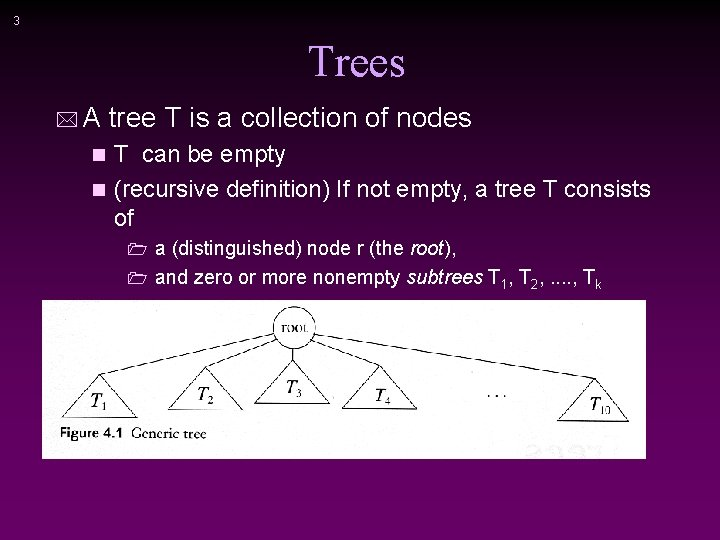3 Trees * A tree T is a collection of nodes T can be empty n (recursive definition) If not empty, a tree T consists of n 1 a (distinguished) node r (the root), 1 and zero or more nonempty subtrees T 1, T 2, . . , Tk4 * Tree can be viewed as a ‘nested’ lists * Tree is also a graph …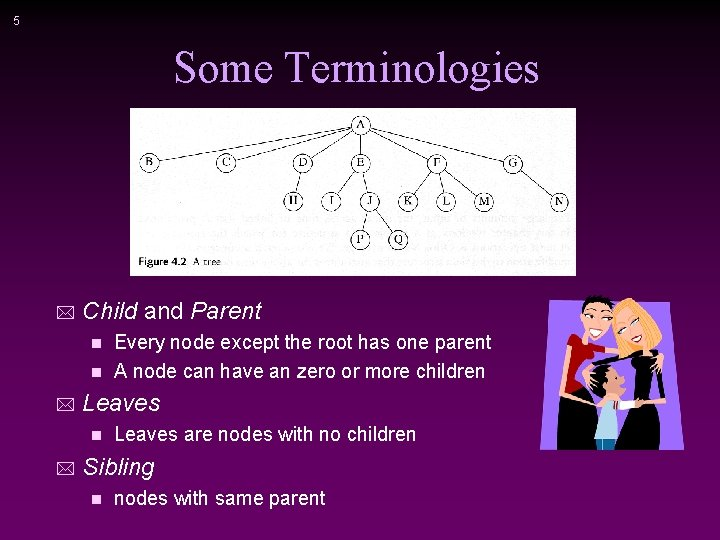5 Some Terminologies * Child and Parent Every node except the root has one parent n A node can have an zero or more children n * Leaves are nodes with no children Sibling n nodes with same parent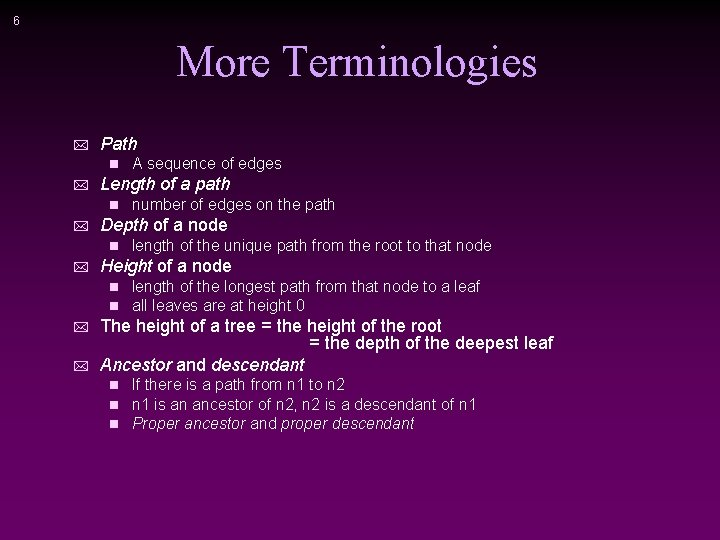6 More Terminologies * Path n * Length of a path n * * length of the unique path from the root to that node Height of a node n n * number of edges on the path Depth of a node n * A sequence of edges length of the longest path from that node to a leaf all leaves are at height 0 The height of a tree = the height of the root = the depth of the deepest leaf Ancestor and descendant n n n If there is a path from n 1 to n 2 n 1 is an ancestor of n 2, n 2 is a descendant of n 1 Proper ancestor and proper descendant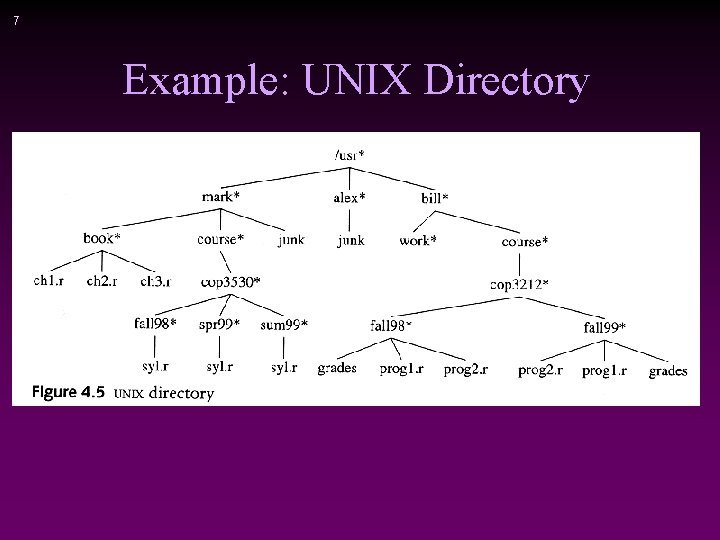7 Example: UNIX Directory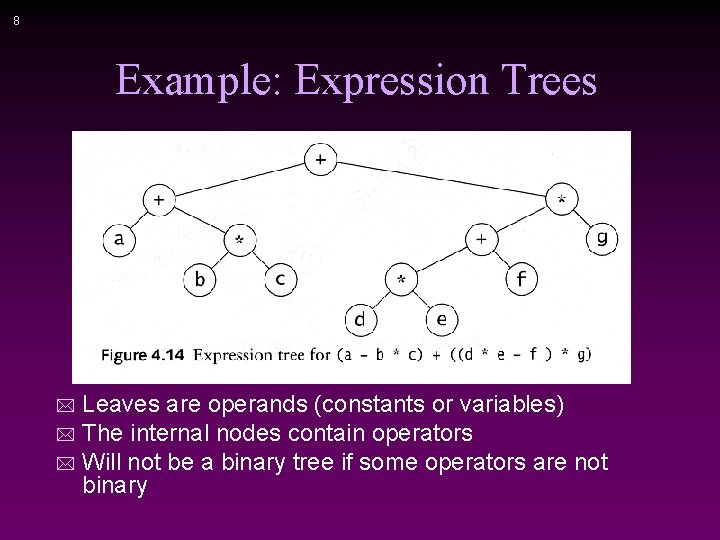8 Example: Expression Trees Leaves are operands (constants or variables) * The internal nodes contain operators * Will not be a binary tree if some operators are not binary *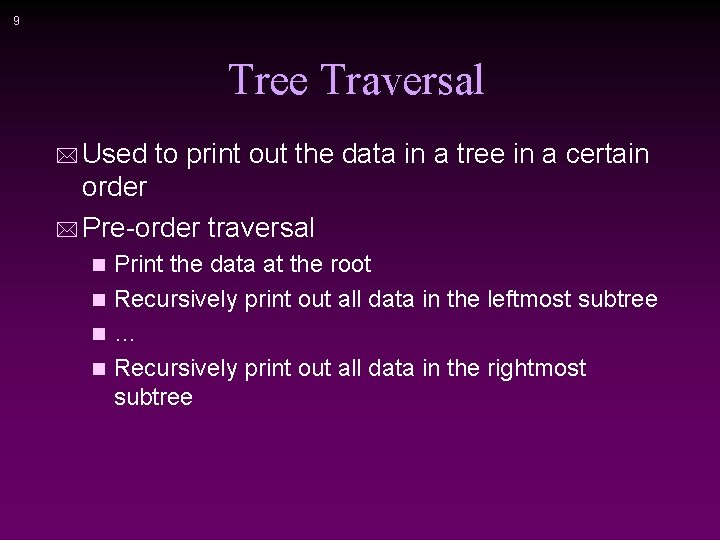9 Tree Traversal * Used to print out the data in a tree in a certain order * Pre-order traversal Print the data at the root n Recursively print out all data in the leftmost subtree n… n Recursively print out all data in the rightmost subtree n10 Preorder, Postorder and Inorder * Preorder traversal node, left, right n prefix expression n 1 ++a*bc*+*defg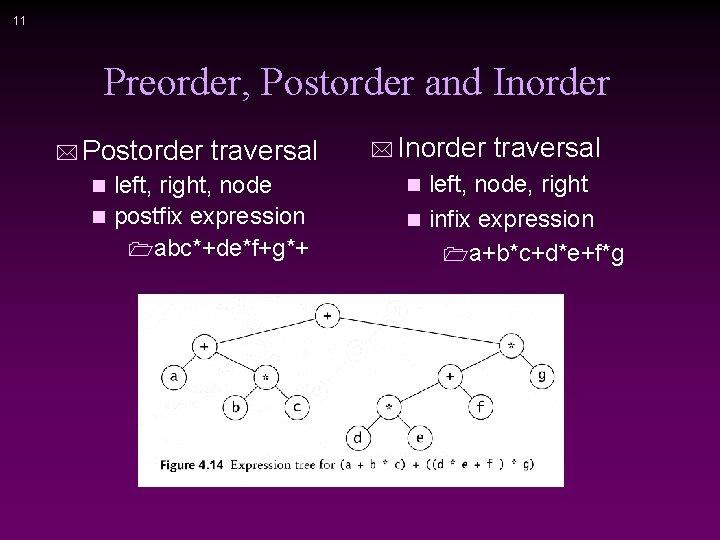11 Preorder, Postorder and Inorder * Postorder traversal left, right, node n postfix expression 1 abc*+de*f+g*+ n * Inorder traversal left, node, right n infix expression 1 a+b*c+d*e+f*g n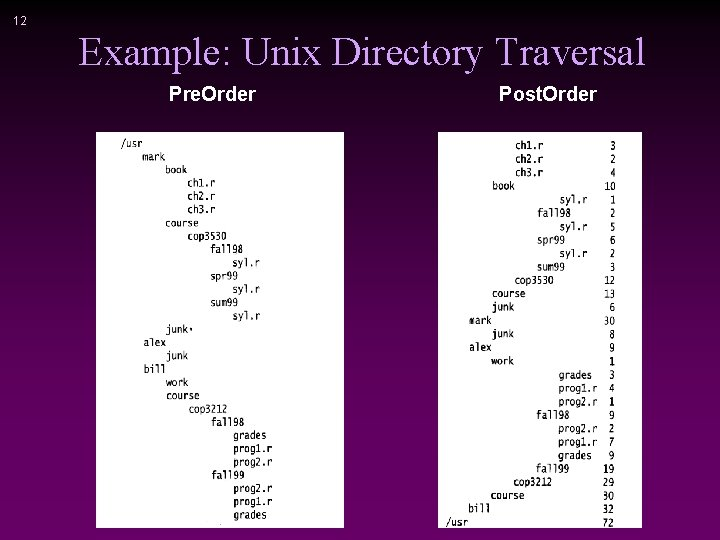12 Example: Unix Directory Traversal Pre. Order Post. Order13 Preorder, Postorder and Inorder Pseudo Code14 Binary Trees * A tree in which no node can have more than two children Generic binary tree * The depth of an “average” binary tree is considerably smaller than N, even though in the worst case, the depth can be as large as N – 1. Worst-case binary tree15 Convert a Generic Tree to a Binary Tree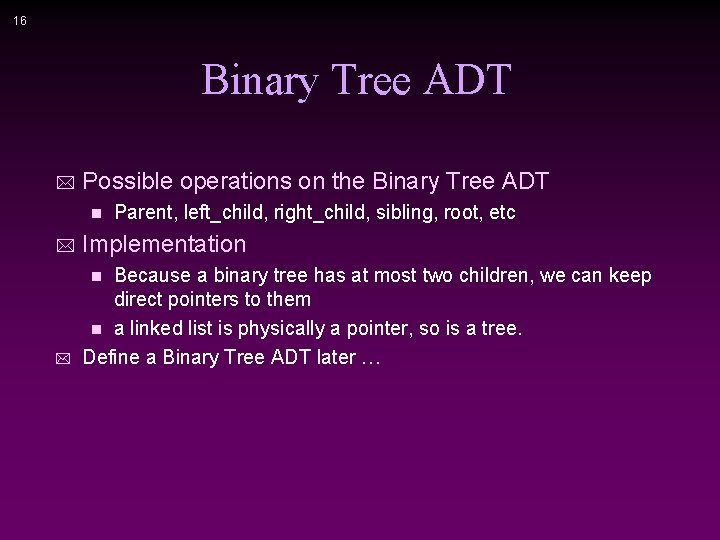16 Binary Tree ADT * Possible operations on the Binary Tree ADT n Parent, left_child, right_child, sibling, root, etc * Implementation * Because a binary tree has at most two children, we can keep direct pointers to them n a linked list is physically a pointer, so is a tree. Define a Binary Tree ADT later … n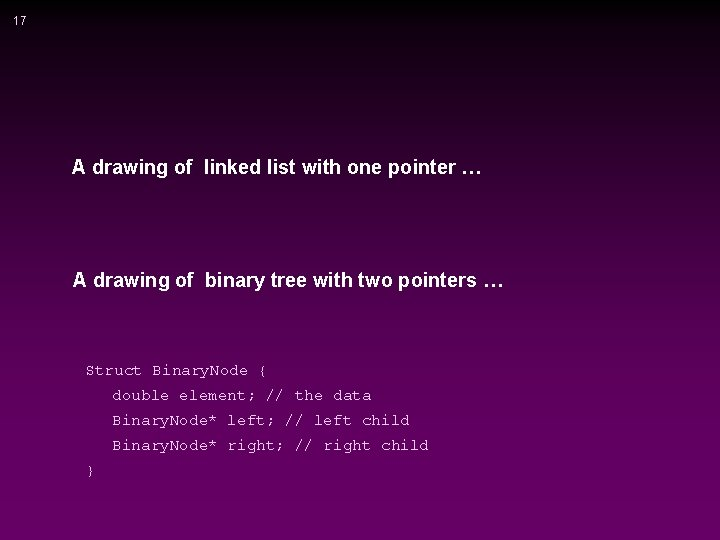17 A drawing of linked list with one pointer … A drawing of binary tree with two pointers … Struct Binary. Node { double element; // the data Binary. Node* left; // left child Binary. Node* right; // right child }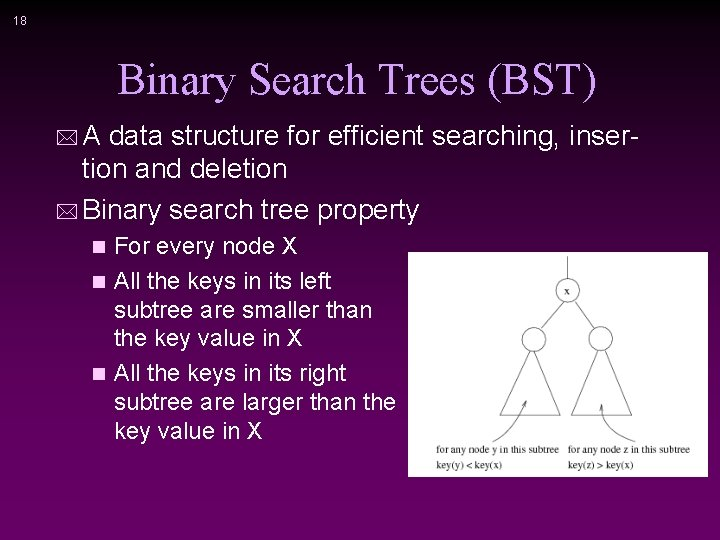18 Binary Search Trees (BST) * A data structure for efficient searching, inser- tion and deletion * Binary search tree property For every node X n All the keys in its left subtree are smaller than the key value in X n All the keys in its right subtree are larger than the key value in X n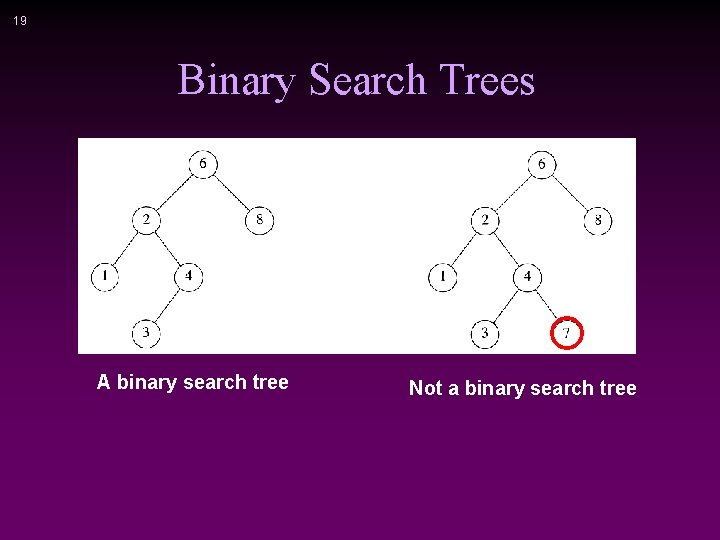19 Binary Search Trees A binary search tree Not a binary search tree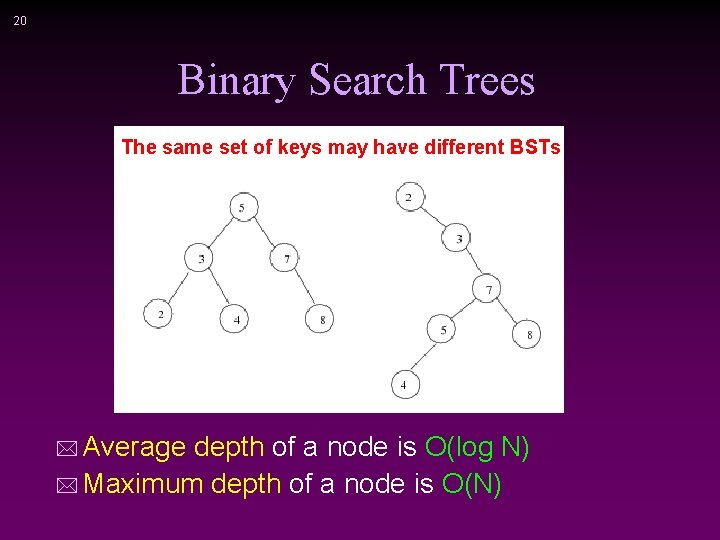20 Binary Search Trees The same set of keys may have different BSTs * Average depth of a node is O(log N) * Maximum depth of a node is O(N)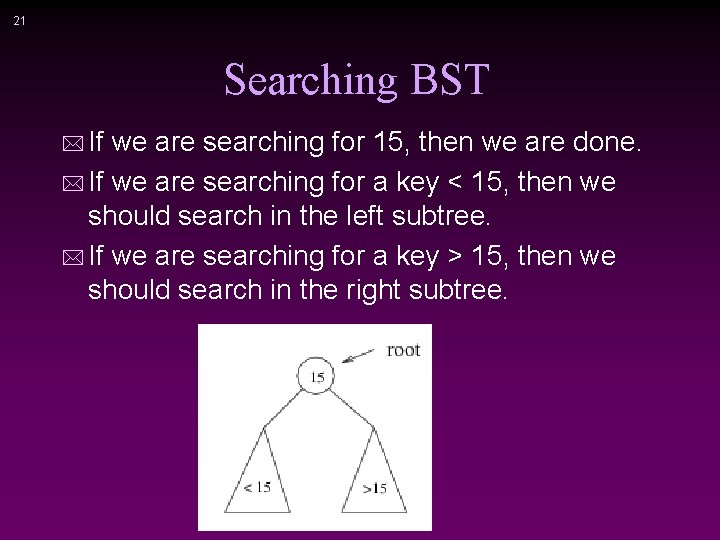21 Searching BST * If we are searching for 15, then we are done. * If we are searching for a key < 15, then we should search in the left subtree. * If we are searching for a key > 15, then we should search in the right subtree.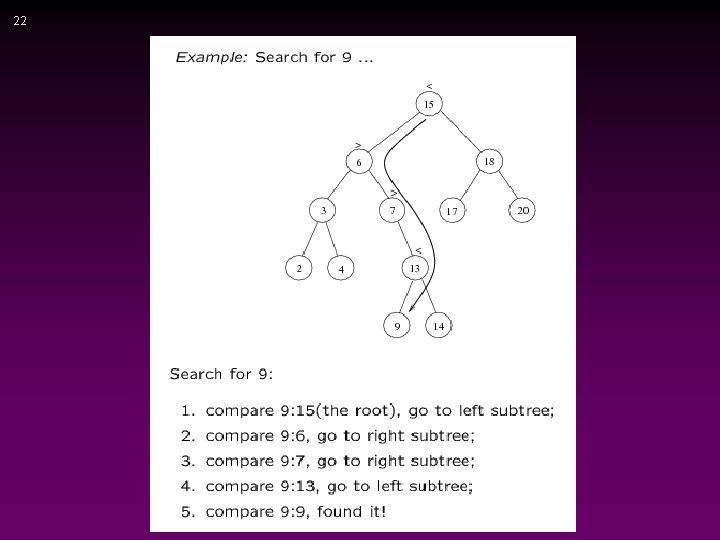2223 Searching (Find) * Find X: return a pointer to the node that has key X, or NULL if there is no such node find(const double x, Binary. Node* t) const * Time complexity: O(height of the tree)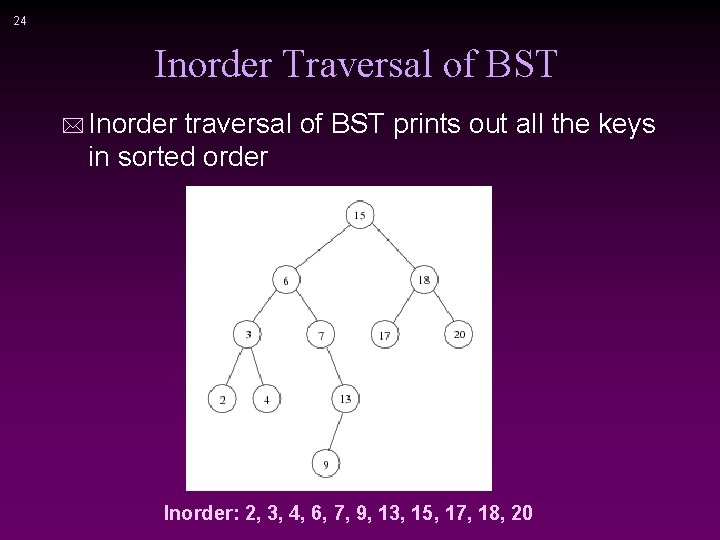24 Inorder Traversal of BST * Inorder traversal of BST prints out all the keys in sorted order Inorder: 2, 3, 4, 6, 7, 9, 13, 15, 17, 18, 20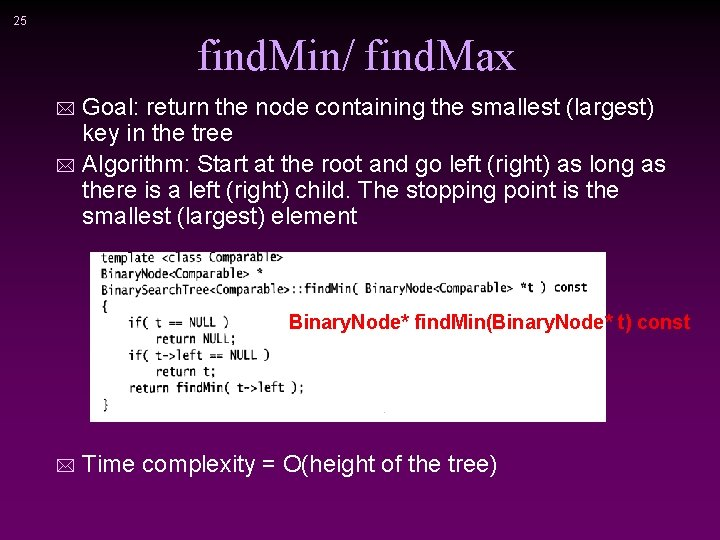25 find. Min/ find. Max Goal: return the node containing the smallest (largest) key in the tree * Algorithm: Start at the root and go left (right) as long as there is a left (right) child. The stopping point is the smallest (largest) element * Binary. Node* find. Min(Binary. Node* t) const * Time complexity = O(height of the tree)26 Insertion Proceed down the tree as you would with a find * If X is found, do nothing (or update something) * Otherwise, insert X at the last spot on the path traversed * * Time complexity = O(height of the tree)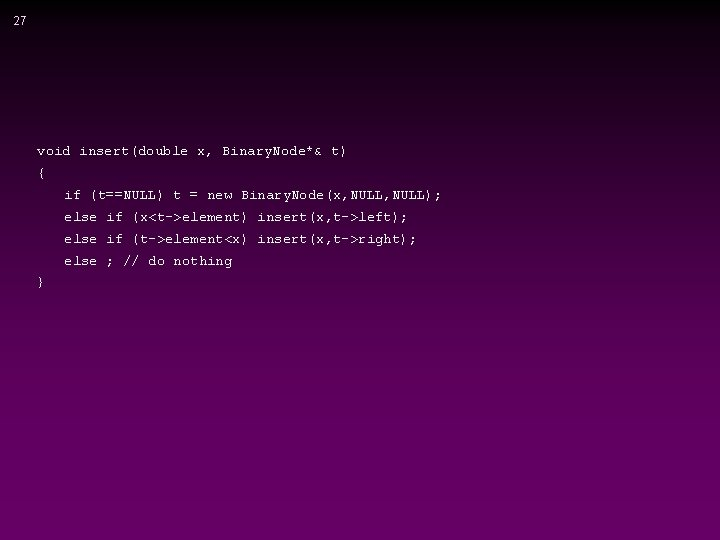27 void insert(double x, Binary. Node*& t) { if (t==NULL) t = new Binary. Node(x, NULL); else if (x<t->element) insert(x, t->left); else if (t->element<x) insert(x, t->right); else ; // do nothing }28 Deletion * When we delete a node, we need to consider how we take care of the children of the deleted node. n This has to be done such that the property of the search tree is maintained.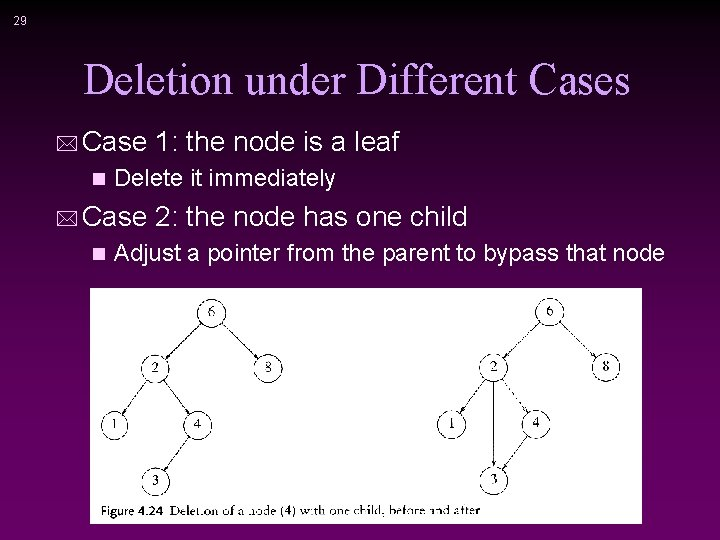29 Deletion under Different Cases * Case 1: the node is a leaf n Delete it immediately * Case 2: the node has one child n Adjust a pointer from the parent to bypass that node30 Deletion Case 3 * Case 3: the node has 2 children Replace the key of that node with the minimum element at the right subtree n Delete that minimum element n 1 Has either no child or only right child because if it has a left child, that left child would be smaller and would have been chosen. So invoke case 1 or 2. * Time complexity = O(height of the tree)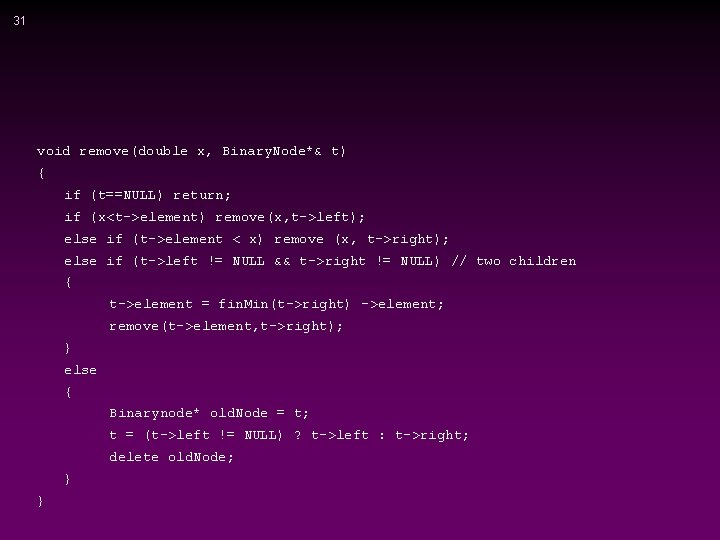31 void remove(double x, Binary. Node*& t) { if (t==NULL) return; if (x<t->element) remove(x, t->left); else if (t->element < x) remove (x, t->right); else if (t->left != NULL && t->right != NULL) // two children { t->element = fin. Min(t->right) ->element; remove(t->element, t->right); } else { Binarynode* old. Node = t; t = (t->left != NULL) ? t->left : t->right; delete old. Node; } }32 Make a binary or BST ADT …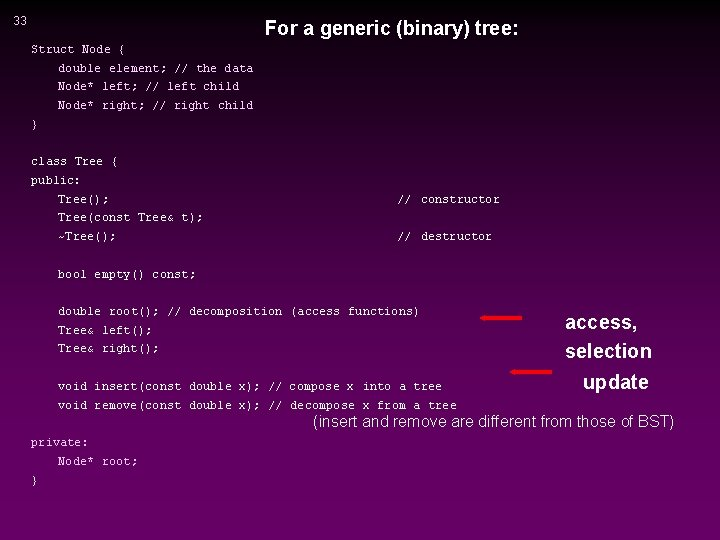33 For a generic (binary) tree: Struct Node { double element; // the data Node* left; // left child Node* right; // right child } class Tree { public: Tree(); // constructor Tree(const Tree& t); ~Tree(); // destructor bool empty() const; double root(); // decomposition (access functions) Tree& left(); Tree& right(); void insert(const double x); // compose x into a tree access, selection update void remove(const double x); // decompose x from a tree (insert and remove are different from those of BST) private: Node* root; }34 For BST tree: Struct Node { double element; // the data Node* left; // left child Node* right; // right child } class BST { public: BST(); // constructor BST(const Tree& t); ~BST(); // destructor bool empty() const; double root(); // decomposition (access functions) BST left(); BST right(); access, selection bool serch(const double x); // search an element void insert(const double x); // compose x into a tree void remove(const double x); // decompose x from a tree update private: Node* root; } BST is for efficient search, insertion and removal, so restricting these functions.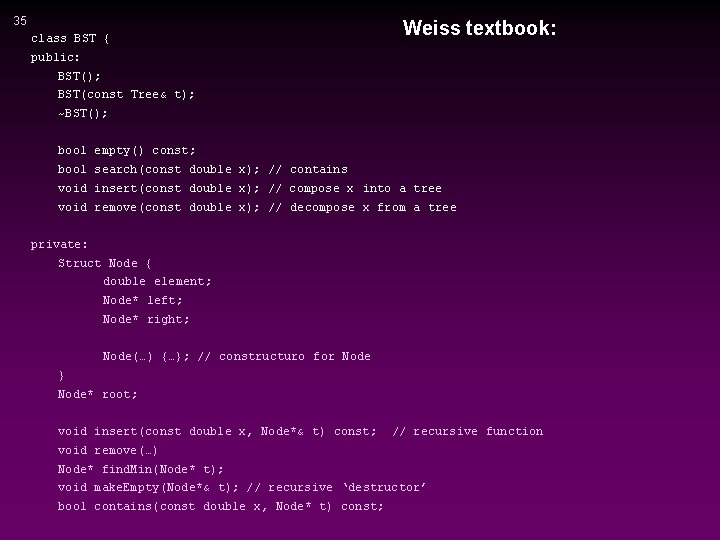35 class BST { Weiss textbook: public: BST(); BST(const Tree& t); ~BST(); bool empty() const; bool search(const double x); // contains void insert(const double x); // compose x into a tree void remove(const double x); // decompose x from a tree private: Struct Node { double element; Node* left; Node* right; Node(…) {…}; // constructuro for Node } Node* root; void insert(const double x, Node*& t) const; // recursive function void remove(…) Node* find. Min(Node* t); void make. Empty(Node*& t); // recursive ‘destructor’ bool contains(const double x, Node* t) const;36 Comments: root, left subtree, right subtree are missing: 1. we can’t write other tree algorithms, is implementation dependent, BUT, 2. this is only for BST (we only need search, insert and remove, may not need other tree algorithms) so it’s two layers, the public for BST, and the private for Binary Tree. 3. it might be defined internally in ‘private’ part (actually it’s implicitly done).37 A public non-recursive member function: void insert(double x) { insert(x, root); } A private recursive member function: void insert(double x, Binary. Node*& t) { if (t==NULL) t = new Binary. Node(x, NULL); else if (x<t->element) insert(x, t->left); else if (t->element<x) insert(x, t->right); else ; // do nothing }